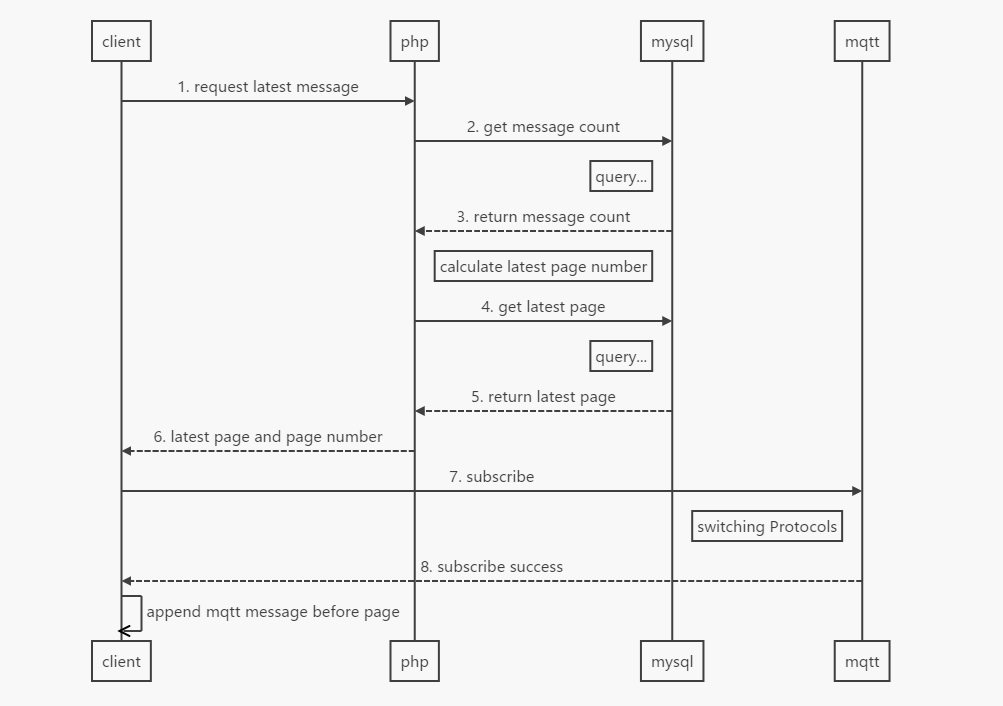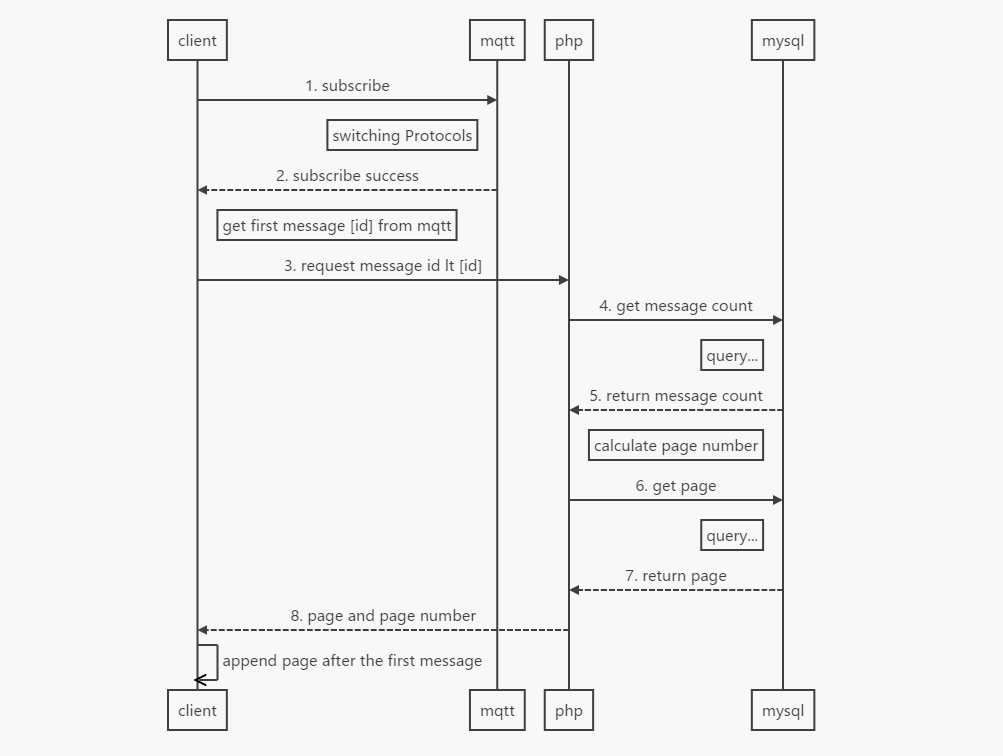分类 PHP 下的文章

高并发下，获取最新评论

But!Naive!测试、分析之后发现，在我们现有前后端交互逻辑（下基本做不到数据不丢失（也可能是我太渣了）。下面是我画的我们现有逻辑的时序图（图1），图为高并发情况下，缓存失效时观众获取最新评论过程，图中省略的cache。在3~4步中，如果新插入数据超出了本页范围，那么新的数据将不会被取到。在5~7步消耗的时间中，用户有可能丢失上百条评论。PHP 变量存储初探

$obj=Obj()::where()->get();//这里是eloquent方法，大概15K条数据 foreach($obj as $k=>$v){
bar();
if($v->user){//eloquent 的has one foo(); } } 这段代码撑爆了单线程128M的内存限制。原因就在循环中$v->user没有释放内存。根据PHP手册对foreach的描述，我们可以得知这里的$v是拷贝赋值，并不是引用赋值。那么为什么每次循环结束的时候，这些内存没有被回收？ 答案在于php存储变量的方式中。PHP中的变量存在一个叫zval的结构体中，我们所用的$a,$b...都只是一个变量的标签，变量真正的值都存在zval容器中。其实每次循环结束$v所占用的内存都已经被回收，但是销毁的 $v只是一个符号，对象真正使用的空间并没有被回收。看完下面这段代码，就应该能很容易的了解到底发生了什么。 echo "<br/>----------- Init a -------------<br/>";$a=new stdClass();
$a->foo=1; xdebug_debug_zval('a'); echo "<br/>----------- Init b ------------<br/>";$b=$a;$b->foo="bar";
xdebug_debug_zval('a');
xdebug_debug_zval('b');

echo "<br/>----------- Unset b ------------<br/>";
unset($b); xdebug_debug_zval('a'); 这段代码将返回： ----------- Init a ------------- a: (refcount=1, is_ref=0), object(stdClass) public 'foo' => (refcount=1, is_ref=0),int 1 ----------- Init b ------------ a: (refcount=2, is_ref=0), object(stdClass) public 'foo' => (refcount=1, is_ref=0),string 'bar' (length=3) b: (refcount=2, is_ref=0), object(stdClass) public 'foo' => (refcount=1, is_ref=0),string 'bar' (length=3) ----------- Unset b ------------ a: (refcount=1, is_ref=0), object(stdClass) public 'foo' => (refcount=1, is_ref=0),string 'bar' (length=3) 这里销毁了$b,但是却并没有将对象销毁掉。所以，在上面的的foreach中，orm对象不停的叠加user中的属性，而一直没有被回收，最终撑爆了内存。

$obj=Obj()::where()->get(); foreach($obj as $k=>$v){
$value = clone$v;//克隆一个对象
bar();
if($value->user){//使用克隆的对象，循环结束立刻销毁 foo(); } } 参考： PHP 中的单双引号 php 果然很有趣 <?php$a1 = null;
$a2 = 'null';$a3 = "null";
$a4 = 0;$a5 = '0';
$a6 = "0";$a7 = array();
$a8 = array(array()); echo empty($a1) ? 'true' : 'false';
echo empty($a2) ? 'true' : 'false'; echo empty($a3) ? 'true' : 'false';
echo empty($a4) ? 'true' : 'false'; echo empty($a5) ? 'true' : 'false';
echo empty($a6) ? 'true' : 'false'; echo empty($a7) ? 'true' : 'false';
echo empty($a8) ? 'true' : 'false'; ?> 得到以下奇怪的结果 文件 是以 php -f test.php执行 cli 是以php -r '$a=null;echo empty(\$a) ? 'true' : 'false';'执行

 null true 1 'null' false 1 "null" false null(这里只是占位，php什么都没有输出) 0 true 1 '0' true 1 "0" true 1 array() true 1 array(array()) false null(这里只是占位，php什么都没有输出)

Walle，我可能的救星

Walle 一个web部署系统工具，配置简单、功能完善、界面流畅、开箱即用！支持git、svn版本管理，支持各种web代码发布，PHP，Python，JAVA等代码的发布、回滚，可以通过web来一键完成。

3月中旬换工作到现在的公司。进来之后简直被震惊到了！！！代码写的各种乱，到处都是漏洞，MySQL玩出了 NoSQL 的感觉。非常庞大的PHP项目，测试和DEV是同一个环境！！！上线居然还是FTP手动上传！！！